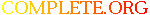Complete.Org: Mailing Lists: Archives: discussion: December 2001: [aclug-L] Nice code and negative memory addresses# [aclug-L] Nice code and negative memory addresses

[Top] [All Lists]

 To: ACLUG Discussion Subject: [aclug-L] Nice code and negative memory addresses From: Lars von dem Ast Date: Sat, 15 Dec 2001 22:41:05 -0600 Reply-to: discussion@xxxxxxxxx

```Here's some nice code (taken from a C text):

/* pmath.c */

#define STRSIZ 20

typedef struct {
char name[STRSIZ];
double diameter;
int moons;
double orbit_time, rotation_time;
} planet_t;

int main(void)
{
planet_t pl = {{"Earth", 12713.5, 1, 1.0, 24.0},
{"Jupiter", 142800.0, 4, 11.9, 9.925}};
int nm = {4, 8, 10, 16, 22};
planet_t *p;
int *np;

p = pl + 1;
np = nm + 1;

printf("sizeof (planet_t) = %d          sizeof (int) = %d\n",
sizeof (planet_t), sizeof (int));
printf("pl = %d                nm = %d\n", pl, nm);
printf(" p = %d (pl + %d)      ", p, (int)p - (int)pl);
printf("np = %d (nm + %d)\n", np, (int)np - (int)nm);
printf(" p - pl = %d\n", p - pl);

}

Okay, here are the results:

sizeof (planet_t) = 48          sizeof (int) = 4
pl = -1073743632                nm = -1073743664
p = -1073743584 (pl + 48)      np = -1073743660 (nm + 4)
p - pl = 1

The pl, p, nm, and np variables should have memory addresses in them, but why
are they negative numbers?

Lb
-- This is the discussion@xxxxxxxxx list.  To unsubscribe,
visit http://tmp2.complete.org/cgi-bin/listargate-aclug.cgi

```